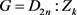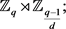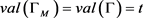﻿ 平方自由阶素数度2-弧正则图

# 平方自由阶素数度2-弧正则图2-Arc-Regular Graphs of Square-Free Order and Prime Valency

Abstract: A graph is called (X,s)-arc regular, if X≤AutΓ is regular on s-arc set. Feng’s paper  determined all one-regular graphs of square-free order and prime valent are Cayley graphs. Quite a lot of works with small valency are known, see . We determined all 2-arc-regular graphs of square-free order and prime valency, where the degreet≡3(mod4).

1. 引言

$Aut\Gamma =PSL\left(2,q\right)$${\left(Aut\Gamma \right)}_{\alpha }={S}_{3}$ ，其中 $q\equiv ±3\left(\mathrm{mod}8\right)$$n=\frac{q\left(q+1\right)\left(q-1\right)}{24}.$

2. 预备引理

1) (二面体) 2-子群；

2) ${A}_{4}$${A}_{7}$

3) $Aut\left(PSL\left(2,q\right)\right)$ 的包含 $PSL\left(2,q\right)$ 的子群，其中 $q\ge 5$ 为奇素数幂。

1)，2nk为平方自由的，k为nk的最小素因子，G为二面体 ${D}_{2n}$ 上的Cayley图；

2) $G=M:X$ ，其中M为平方自由阶的，X为几乎单群且a) $soc\left(G\right)={M}_{11},{M}_{12},{M}_{22},{M}_{23},{M}_{24,}{J}_{1}$

b) $G={A}_{n}$ 或者 ${S}_{n}$ ，其中 $n<3k$

c) $G=PSL\left(2,p\right)$$G=PGL\left(2,p\right)$

d) $soc\left(G\right)=PSL\left(2,{p}^{f}\right)$$f\ge 2$ ，且 ${p}^{f}>9$ ，或者 $k|{p}^{f-1}$ 或者 $f=2$$k|p+1$

e) G为Lie型单群 $GF\left({p}^{f}\right)$$p\le k$ ，或者 $\left[\frac{d}{2}\right]f ，G为d维典型群， $d\ge 3$ 。或者 $2f

$soc\left(G\right)={G}_{2}\left({p}^{f}\right),{D}_{4}\left({p}^{f}\right),{F}_{4}\left({p}^{f}\right),{E}_{6}\left({p}^{f}\right),{E}_{7}\left({p}^{f}\right)$ 。且 $MT=M×T$ 有至多两个轨道并且G为T-边传递图，特别地，如 $T=PSL\left(2,p\right)$ ，则 $M,{T}_{\alpha },k$ 满足[  ，表3]。

1) $Aut\left(\Gamma \right)$ 可解，且 $Aut\left(\Gamma \right)\cong {D}_{2n}:{Z}_{3}$

2) $Aut\left(\Gamma \right)=PSL\left(2,p\right)$ ，其中 $p\ge 19$ 为一素数。

1) N在VG半正则， $X/N\le Aut\left({\Gamma }_{N}\right)$$\left(X/N,s\right)$ -弧正则图，且G为 ${\Gamma }_{N}$ 的一个正规N-覆盖；

2) ${X}_{\alpha }\cong {\left(X/N\right)}_{\delta }$ ，其中 $\alpha \in V\Gamma$$\delta \in V{\Gamma }_{M}$

3) 如果X有一正规子群 $M\subset N$ ，则 ${\Gamma }_{M}$$\left(X/M,s\right)$ -弧正则，且是 ${\Gamma }_{N}$ 的一个正规N/M-覆盖。

$PSL\left(2,q\right)$ 的极大子群是知道的，下面我们给出一个引理，参见  。

1) ${D}_{\frac{2\left(q-1\right)}{d}}$ ，其中 $d=\left(2,q-1\right)$ ，且 $q\ne 5,7,9,11$

2) ${D}_{\frac{2\left(q+1\right)}{d}}$ ，其中  ，且 $q\ne 7,9$

3)4) ${A}_{4}$ ，其中 $q=p=5$ ，或 $q=p=3,13,27,37\left(\mathrm{mod}40\right)$

5) ${S}_{4}$ ，其中 $q\equiv ±1\left(\mathrm{mod}8\right)$

6) ${A}_{5}$ ，其中 $q\equiv ±1\left(\mathrm{mod}10\right)$

7) $PSL\left(2,{p}^{m}\right)$$n/m$ 为奇素数；

8) $PSL\left(2,{p}^{\frac{n}{2}}\right)$ ，n为偶数。

a = (1, 3)(2, 13)(4, 5)(6, 10)(7, 12)(8, 19)(9, 14)(11, 15)(16, 17)(18, 20)；

b = (1, 16, 4)(3, 5, 17)(6, 20, 7)(8, 15, 9)(10, 12, 18)(11, 19, 14)；

c = (1, 18)(2, 8)(3, 20)(4, 16)(5, 17)(6, 11)(7, 9)(10, 15)(12, 14)(13, 19)；

e = (3, 16)(4, 20)(5, 26)(6, 9)(7, 28)(8, 14)(10, 18)(11, 12)(13, 29)(15, 23)(17, 30)(19, 25)(21, 22)(24, 27)；

f = (1, 14, 8)(2, 7, 28)(3, 27, 10)(4, 9, 13)(5, 12, 21)(6, 20, 29)(11, 26, 22)(15, 30, 19)(16, 18, 24)(17, 23, 25)；

g1 = (1, 2)(3, 25)(4, 7)(5, 29)(6, 15)(8, 17)(9, 23)(10, 27)(13, 26)(14, 30)(16, 19)(18, 24)(20, 28)(21, 22)；

g2 = (1, 2)(4, 21)(5, 11)(6, 25)(7, 23)(8, 14)(9, 19)(10, 30)(12, 26)(13, 24)(15, 28)(17, 18)(20, 22)(27, 29)。

$|H:H\cap {H}^{{g}_{1}}|=|H:H\cap {H}^{{g}_{2}}|=3$ ，因此 $\mathrm{Cos}\left(G,H,H{g}_{1}H\right)$$\mathrm{Cos}\left(G,H,H{g}_{2}H\right)$$\left(G,2\right)$ -弧正则3度图。更多的，这两个图是同构意义下，以 $PSL\left(2,29\right)$ 作为全自同构群的唯一的两个不同构的2-弧正则3度图。

3. 定理证明

( ${\left({\Delta }_{1}^{g}\right)}^{{g}^{-1}}={\Delta }_{2}={\Delta }_{1}$ ，矛盾。)而且，因为A在VG上传递，所以 $|A:{A}^{+}|=2$ 。由Frattini论断， ${A}^{+}=M{A}_{\alpha }{}^{+}=M{A}_{\alpha }$ ，则 ${A}^{+}/M=M{A}_{\alpha }/M\cong {A}_{\alpha }/{A}_{\alpha }\cap M$ 为可解的，且M可解，故 ${A}^{+}$ 可解。又因为 $|A:{A}^{+}|=2$${A}^{+}⊲A$ ，故A可解。矛盾。

$|V{\Gamma }_{M}|=\frac{2n}{|M|}$ 为平方自由的，。设 $\alpha \in V\Gamma$$\delta \in V{\Gamma }_{M}$$\stackrel{¯}{A}=A/M$ ，则

${A}_{\alpha }\cong {\stackrel{¯}{A}}_{\alpha }\cong {ℤ}_{t}:{ℤ}_{t-1}$ 。设 $\stackrel{¯}{{A}_{\sigma }}\le Y$ ，Y为 $PSL\left(2,q\right)$ 的极大子群，由断言，我们知道q为奇素数，所以由推论得 $t=3$ 。最后，因为A不可解且 ${|A|}_{2}=4$ ，由引理2.3，我们得到 $\left(A,{A}_{\alpha }\right)=\left(PSL\left(2,q\right),{S}_{3}\right)$ ，且 $q\ge 19$ 为奇素数。因为 $|A:{A}_{\alpha }|=\frac{q\left(q-1\right)\left(q+1\right)}{24}=2n$ ，其中n是平方自由的奇素数，所以 $8|{q}^{2}-1$$16\nmid {q}^{2}-1$$8\nmid q+1$

$8\nmid q-1$ ，得 $q\equiv ±3\left(\mathrm{mod}8\right)$$n=\frac{q\left(q+1\right)\left(q-1\right)}{24}$ 定理得证。

 Feng, Y.Q. and Li, Y.T. (2011) One-Regular Graphs of Square-Free Order of Prime Valency. European Journal of Combinatorics, 32, 261-275.
https://doi.org/10.1016/j.ejc.2010.10.002

 Fang, X., Wang, J. and Xu, M.Y. (2002) On 1-Arc-Regular Graphs. European Journal of Combinatorics, 23, 785-791.
https://doi.org/10.1006/eujc.2002.0579

 Feng, Y.Q. and Kwak, J.H. (2004) One-Regular Cubic Graphs of Order a Small Number Times a Prime or Prime Square. Journal of the Australian Mathematical Society, 76, 345-356.
https://doi.org/10.1017/S1446788700009903

 Feng, Y.Q. and Kwak, J.H. (2007) Cubic Symmetric Graphs of Order a Small Number Times a Prime or a Prime Square. Journal of Combinatorial Theory, Series B, 97, 627-646.
https://doi.org/10.1016/j.jctb.2006.11.001

 Pan, J.M., Ding, S.Y. and Liu, Y. (2014) Finite Arc-Regular Graphs of Prime Valency. Scientia Sinica Mathematica, 44, 307-315.
https://doi.org/10.1360/012014-1

 Li, C.H., Lu, Z.P. and Wang, C.X. (2015) On Edge-Transitive Graphs of Square-Free Order. European Journal of Combinatorics, 22, 41-46.
https://doi.org/10.1016/j.ejc.2014.10.005

 Ling, B. and Lou, B.G. (2017) Arc-Transitive Cubic Graphs of Order Four Times an Odd Square-Free Integer. Journal of Algebra and Its Applications, 16, 213-225.
https://doi.org/10.1142/S0219498817502139

 Bosma, W., Cannon, J. and Playoust, C. (1997) The MAGMA Algebra System I: The User Language. Journal of Symbolic Computation, 24, 235-265.
https://doi.org/10.1006/jsco.1996.0125

 Biggs, N. (1992) Algebraic Graph Theory. 2nd Edition, Cambridge University Press, New York.

 Dickson, L.E. (1958) Linear Groups with an Expositions of the Galois Field Theory. Dover.

Top